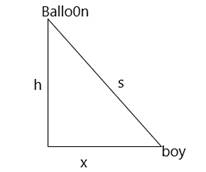# Tofind: how fast the distance between the boy and balloon increases at 3s later.### Single Variable Calculus: Concepts...

4th Edition
James Stewart
Publisher: Cengage Learning
ISBN: 9781337687805### Single Variable Calculus: Concepts...

4th Edition
James Stewart
Publisher: Cengage Learning
ISBN: 9781337687805

#### Solutions

Chapter 4, Problem 37RE
To determine

## Tofind:how fast the distance between the boy and balloon increases at 3s later.

Expert Solution

It increases at 13ft/s .

### Explanation of Solution

Given:

dhdt=5ft/sec

dxdt=15ft/sec

Concept used:

According to the Pythagoras theorem:

s2=x2+h2 .

If d2ydx2>0. the concave will be open upward and local minima can be found.

If d2ydx2<0. the concave will open downward and local maxima can be found.

According to the Pythagoras theorem:

s2=x2+h2 .

Calculation:

Let the figure of the question be:According to the given data: dhdt=5ft/sec and dxdt=15ft/sec .

According to the Pythagoras theorem:

s2=x2+h2 .

Differentiating with respect to t:

2sdsdt=2xdxdt+2hdhdt .

sdsdt=xdxdt+hdhdt .

dsdt=1s(xdxdt+hdhdt) .

To find the length at 3 seconds:

x=15fts3s=45ft .

h=5fts.3s+45=60ft .

s=452+602=5625=75ft .

Plug in known value for the moment in time:

dsdt=1s(xdxdt+hdhdt)

dsdt=175(45(15)+60(5)) .

97575

dsdt=13ft/s

Hence it increases at 13ft/s .

### Have a homework question?

Subscribe to bartleby learn! Ask subject matter experts 30 homework questions each month. Plus, you’ll have access to millions of step-by-step textbook answers!